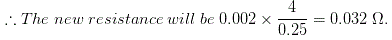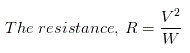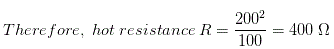# MCQs on Electric Circuits

##### Page 25 of 63. Go to page 1 2 3 4 5 6 7 8 9 10 11 12 13 14 15 16 17 18 19 20 21 22 23 24 25 26 27 28 29 30 31 32 33 34 35 36 37 38 39 40 41 42 43 44 45 46 47 48 49 50 51 52 53 54 55 56 57 58 59 60 61 62 63
01․ A 10Ω resistor is stretched to increase its length double. Its resistance will now be
40 Ω.
20 Ω.
10 Ω.
5 Ω.

The resistance of a conductor is directly proportional to its length and inversely proportional to its cross-sectional area. As the wire is stretched to its double length, its cross-sectional area will become half, hence, the resistance of the stretched wire will become 4 times.

02․ Specific resistance is measured in
mho.
ohm.
ohm - cm.
ohm/cm.

The resistance R = ρl / a. Where R is the resistance of any substance in ohm, ρ is the specific resistance of the material of that substance, l and a are length in cm and cross - sectional area in cm2 of that substance respectively. Therefore,  ρ = Ra / l and its unit may be ohm - cm2/cm or ohm - cm.

03․ A wire of resistance R has it length and cross - section both doubled. Its resistance will become
0.5R.
R.
2R.
4R.

The resistance of a conductor is directly proportional to its length and inversely proportional to its cross - sectional area. As the length and cross - sectional area both have become double, there will be no change in the resistance of the wire.

04․ A cube of material of side 1 cm has a resistance of 0.002 Ω between its opposite faces. If the same volume of the material has a length of 4 cm and a uniform cross - section, the resistance of this length will be
0.128 Ω.
0.064 Ω.
0.032 Ω.
0.016 Ω.

Here, the cube of the material of side 1 cm has a resistance of 0.002 Ω between its opposite faces that means the resistivity of the material is 0.002 Ω. Now the length of the material has become 4 cm, hence for same volume 1 cm3 the cross - sectional area of the material will be 1/4 or 0.25 cm2.05․ Resistance of which material does not change with change in temperature?
Platinum.
Metal alloys constantan and manganin.
Brass.
Tungsten.

Resistance remains constant with change in temperature in the metal alloy of constantan and manganin. This material has temperature coefficient equal to zero. It is used for precision wire wound resistors, which does not show change in resistance when the temperature is increased.

06․ The hot resistance of a tungsten lamp is about 10 times the cold resistance. Accordingly, cold resistance of a 100 W, 200 V lamp will be
400 Ω.
40 Ω.
4 Ω.
800 Ω.Where, V is supply voltage and W is wattage rating of the lamp. Here, V = 200 V and W = 100 W,∴ Cold resistance of the lamp is 400 ÷ 10 = 40 Ω.

07․ For same voltage, the ratio resistance of 100 W lamp to resistance of 25 W lamp is
16.
4.
1/4.
1.Where, V is supply voltage and W is wattage rating of the lamp. From, above equation it is clear that, for fixed voltage source, resistance of an electric lamp is inversely proportional to its wattage rating. That means, ratio of resistance will be just reverse of their ratio of wattage rating.

08․ Which of the following has least resistivity?
Copper.
Mercury.
Aluminium.

MaterialsResistivity at 20oC
Copper1.68 × 10- 8 Ω - m
Lead22 × 10- 8 Ω - m
Mercury98 × 10- 8 Ω - m
Aluminium2.65 × 10 Ω - m

09․ Which of the following lamp has least resistance ?
200 W, 220 V.
100 W, 220 V.
60 W, 220 V.
25 W, 220 V.Where, V is supply voltage and W is wattage rating of the lamp. From, above equation it is clear that, for fixed voltage source , resistance of an electric lamp is inversely proportional to its wattage rating. Here, 200 W, 220 V lamp has highest wattage rating, therefore its resistance will be least.

10․ 200 resistors of 200 Ω each are connected in parallel. Their equivalent resistance will be
1 Ω.
200 Ω.
400 Ω.
4 kΩ.

If N numbers of resistors of R Ω each are connected in parallel. Their equivalent resistance will be R ÷ N.

<<<2324252627>>>